Testing for Heteroscedasticity

The regression model is specified as, where the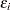’s are identically and independently distributed:and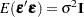. If the’s are not independent or their variances are not constant, the parameter estimates are unbiased, but the estimate of the covariance matrix is inconsistent.

In the case of heteroscedasticity, if the regression data are from a simple random sample, then White (1980), showed that matrix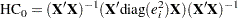whereis an asymptotically consistent estimate of the covariance matrix. MacKinnon and White (1985) introduced three alternative heteroscedasticity-consistent covariance matrix estimators that are all asymptotically equivalent to the estimator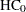but that typically have better small sample behavior. These estimators labeled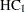,, andare defined as follows:whereis the number of observations andis the number of regressors including the intercept.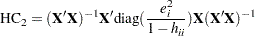where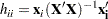is the leverage of theth observation.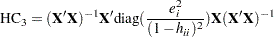Long and Ervin (2000) studied the performance of these estimators and recommend using theestimator if the sample size is less than 250.

You can use the HCCMETHOD=0,1,2, or 3 in the MODEL statement to select a heteroscedasticity-consistent covariance matrix estimator, withbeing the default. The ACOV option in the MODEL statement displays the heteroscedasticity-consistent covariance matrix estimator in effect and adds heteroscedasticity-consistent standard errors, also known as White standard errors, to the parameter estimates table. If you specify the HCC or WHITE option in the MODEL statement, but do not also specify the ACOV option, then the heteroscedasticity-consistent standard errors are added to the parameter estimates table but the heteroscedasticity- consistent covariance matrix is not displayed.

The SPEC option performs a model specification test. The null hypothesis for this test maintains that the errors are homoscedastic and independent of the regressors and that several technical assumptions about the model specification are valid. For details, see theorem 2 and assumptions 1–7 of White (1980). When the model is correctly specified and the errors are independent of the regressors, the rejection of this null hypothesis is evidence of heteroscedasticity. In implementing this test, an estimator of the average covariance matrix (White 1980, p. 822) is constructed and inverted. The nonsingularity of this matrix is one of the assumptions in the null hypothesis about the model specification. When PROC REG determines this matrix to be numerically singular, a generalized inverse is used and a note to this effect is written to the log. In such cases, care should be taken in interpreting the results of this test.

When you specify the SPEC, ACOV, HCC, or WHITE option in the MODEL statement, tests listed in the TEST statement are performed with both the usual covariance matrix and the heteroscedasticity-consistent covariance matrix requested with the HCCMETHOD= option. Tests performed with the consistent covariance matrix are asymptotic. For more information, refer to White (1980).

Both the ACOV and SPEC options can be specified in a MODEL or PRINT statement.